1 de Jun de 2023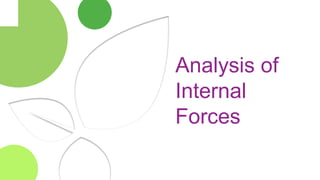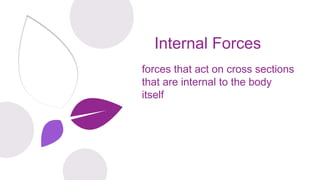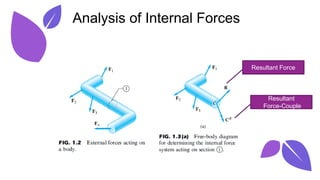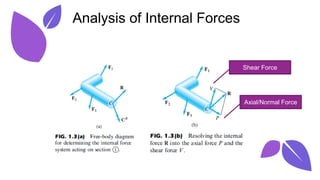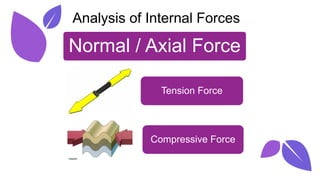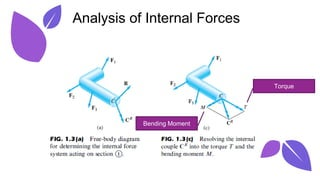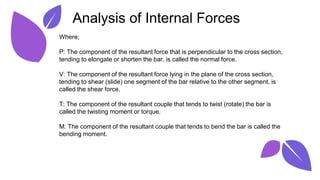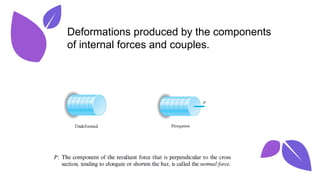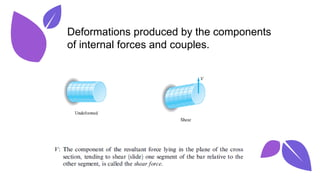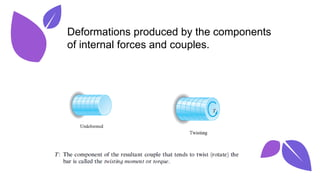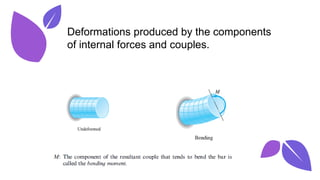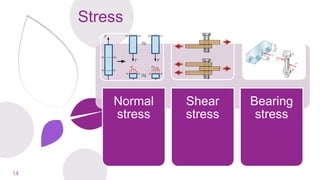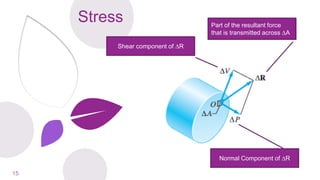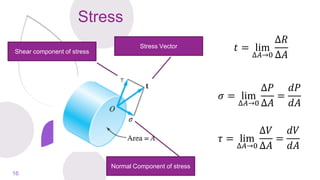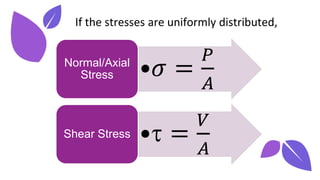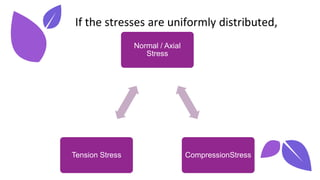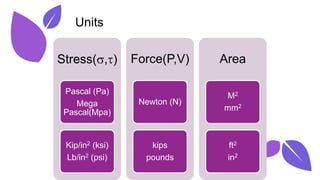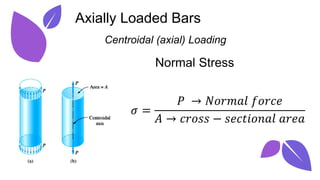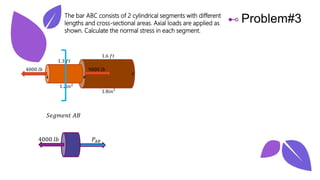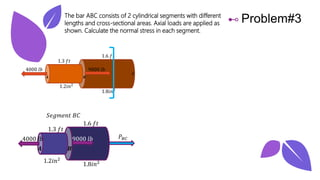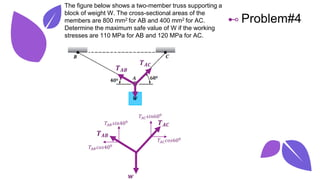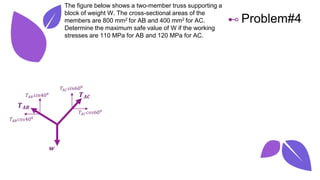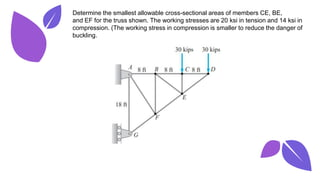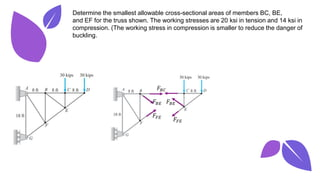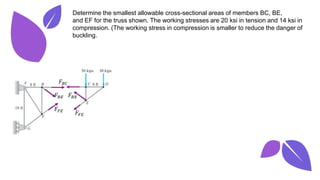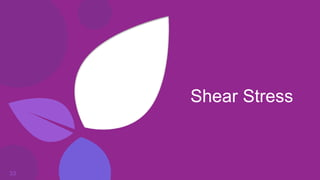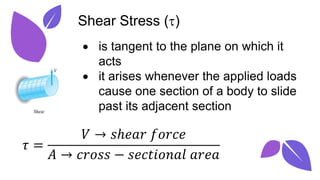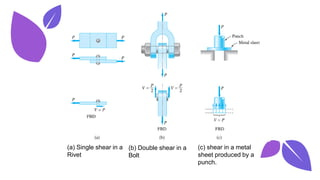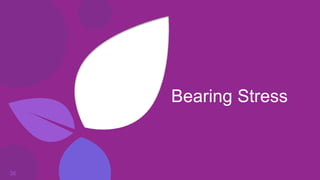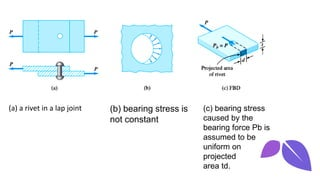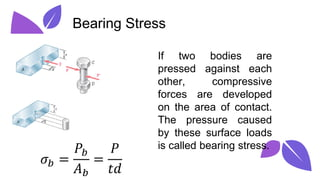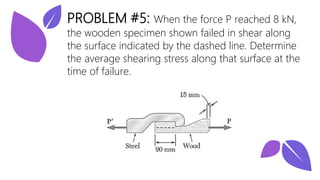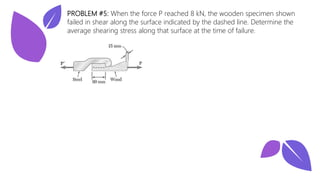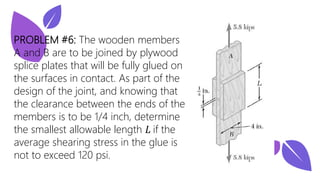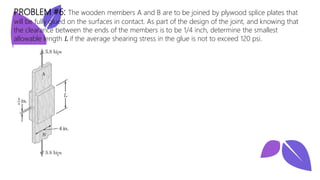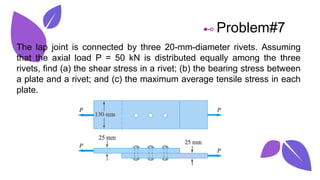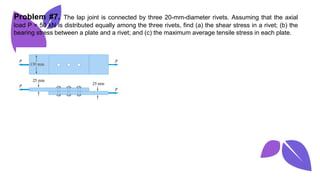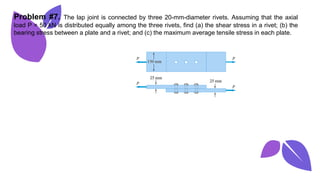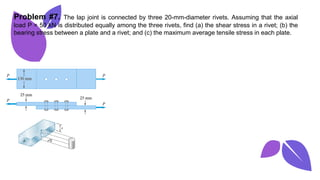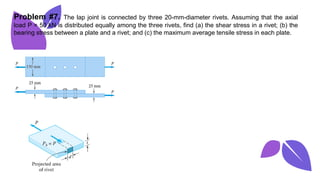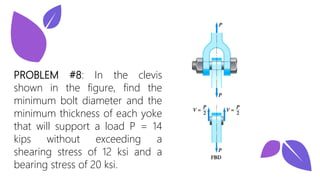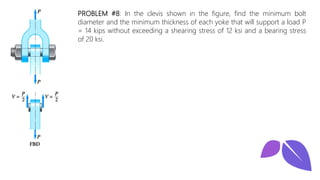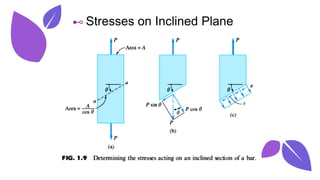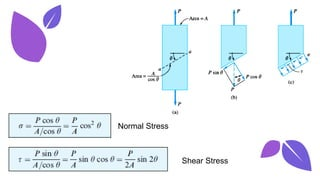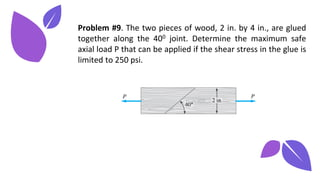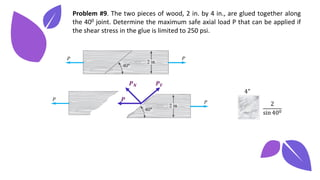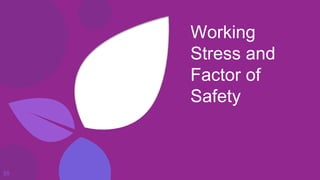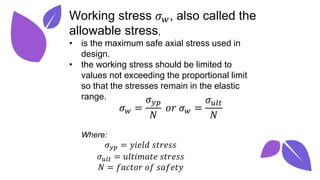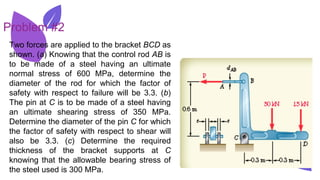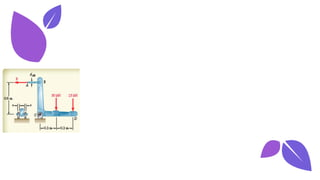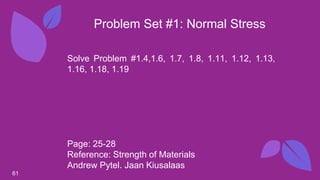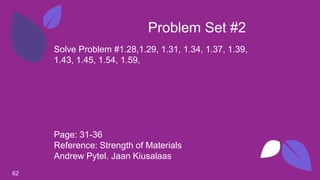1 de 62

• 1. Internal Forces Stress Lesson #1
• 2. Analysis of Internal Forces
• 3. Internal Forces forces that act on cross sections that are internal to the body itself
• 4. 4 Analysis of Internal Forces Resultant Force Resultant Force-Couple
• 5. 5 Analysis of Internal Forces Shear Force Axial/Normal Force
• 6. 6 Analysis of Internal Forces Normal / Axial Force Tension Force Compressive Force
• 7. 7 Analysis of Internal Forces Torque Bending Moment
• 8. 8 Analysis of Internal Forces Where; P: The component of the resultant force that is perpendicular to the cross section, tending to elongate or shorten the bar, is called the normal force. V: The component of the resultant force lying in the plane of the cross section, tending to shear (slide) one segment of the bar relative to the other segment, is called the shear force. T: The component of the resultant couple that tends to twist (rotate) the bar is called the twisting moment or torque. M: The component of the resultant couple that tends to bend the bar is called the bending moment.
• 9. 9 Deformations produced by the components of internal forces and couples.
• 10. 10 Deformations produced by the components of internal forces and couples.
• 11. 11 Deformations produced by the components of internal forces and couples.
• 12. 12 Deformations produced by the components of internal forces and couples.
• 13. Stress 13
• 14. Stress 14 Normal stress Shear stress Bearing stress
• 15. Stress 15 Shear component of R Normal Component of R Part of the resultant force that is transmitted across A
• 16. Stress 16 Shear component of stress Normal Component of stress Stress Vector 𝑡 = lim ∆𝐴→0 ∆𝑅 ∆𝐴 𝜎 = lim ∆𝐴→0 ∆𝑃 ∆𝐴 = 𝑑𝑃 𝑑𝐴 𝜏 = lim ∆𝐴→0 ∆𝑉 ∆𝐴 = 𝑑𝑉 𝑑𝐴
• 17. 17 If the stresses are uniformly distributed, •𝜎 = 𝑃 𝐴 Normal/Axial Stress • = 𝑉 𝐴 Shear Stress
• 18. 18 If the stresses are uniformly distributed, Normal / Axial Stress CompressionStress Tension Stress
• 19. 19 Units Stress(,) Pascal (Pa) Mega Pascal(Mpa) Kip/in2 (ksi) Lb/in2 (psi) Force(P,V) Newton (N) kips pounds Area M2 mm2 ft2 in2
• 20. 20 Axially Loaded Bars Centroidal (axial) Loading Normal Stress 𝜎 = 𝑃 → 𝑁𝑜𝑟𝑚𝑎𝑙 𝑓𝑜𝑟𝑐𝑒 𝐴 → 𝑐𝑟𝑜𝑠𝑠 − 𝑠𝑒𝑐𝑡𝑖𝑜𝑛𝑎𝑙 𝑎𝑟𝑒𝑎
• 21. 21 ⊷ Problem#3 The bar ABC consists of 2 cylindrical segments with different lengths and cross-sectional areas. Axial loads are applied as shown. Calculate the normal stress in each segment. 4000 𝑙𝑏 9000 𝑙𝑏 1.3 𝑓𝑡 1.6 𝑓𝑡 1.2𝑖𝑛2 1.8𝑖𝑛2 𝑨 𝑩 𝑪
• 22. 22 ⊷ Problem#3 The bar ABC consists of 2 cylindrical segments with different lengths and cross-sectional areas. Axial loads are applied as shown. Calculate the normal stress in each segment. 4000 𝑙𝑏 𝑃𝐴𝐵 𝑆𝑒𝑔𝑚𝑒𝑛𝑡 𝐴𝐵
• 23. 23 ⊷ Problem#3 The bar ABC consists of 2 cylindrical segments with different lengths and cross-sectional areas. Axial loads are applied as shown. Calculate the normal stress in each segment. 𝑃𝐵𝐶 𝑆𝑒𝑔𝑚𝑒𝑛𝑡 𝐵𝐶 4000 𝑙𝑏 9000 𝑙𝑏 1.3 𝑓𝑡 1.6 𝑓𝑡 1.2𝑖𝑛2 1.8𝑖𝑛2 𝑨 𝑩
• 24. 24 ⊷ Problem#4 The figure below shows a two-member truss supporting a block of weight W. The cross-sectional areas of the members are 800 mm2 for AB and 400 mm2 for AC. Determine the maximum safe value of W if the working stresses are 110 MPa for AB and 120 MPa for AC.
• 25. 25 ⊷ Problem#4 The figure below shows a two-member truss supporting a block of weight W. The cross-sectional areas of the members are 800 mm2 for AB and 400 mm2 for AC. Determine the maximum safe value of W if the working stresses are 110 MPa for AB and 120 MPa for AC. 𝑻𝑨𝑩 𝑻𝑨𝑪 𝑻𝑨𝑩 𝑻𝑨𝑪 𝒘 𝑇𝐴𝐵𝑠𝑖𝑛400 𝑇𝐴𝐵𝑐𝑜𝑠400 𝑇𝐴𝐶𝑠𝑖𝑛600 𝑇𝐴𝐶𝑐𝑜𝑠600
• 26. 26 ⊷ Problem#4 The figure below shows a two-member truss supporting a block of weight W. The cross-sectional areas of the members are 800 mm2 for AB and 400 mm2 for AC. Determine the maximum safe value of W if the working stresses are 110 MPa for AB and 120 MPa for AC. 𝑻𝑨𝑩 𝑻𝑨𝑪 𝒘 𝑇𝐴𝐵𝑠𝑖𝑛400 𝑇𝐴𝐵𝑐𝑜𝑠400 𝑇𝐴𝐶𝑠𝑖𝑛600 𝑇𝐴𝐶𝑐𝑜𝑠600
• 27. 27 ⊷ Problem#4 The figure below shows a two-member truss supporting a block of weight W. The cross-sectional areas of the members are 800 mm2 for AB and 400 mm2 for AC. Determine the maximum safe value of W if the working stresses are 110 MPa for AB and 120 MPa for AC. 𝑻𝑨𝑩 𝑻𝑨𝑪 𝒘 𝑇𝐴𝐵𝑠𝑖𝑛400 𝑇𝐴𝐵𝑐𝑜𝑠400 𝑇𝐴𝐶𝑠𝑖𝑛600 𝑇𝐴𝐶𝑐𝑜𝑠600
• 28. 28 Determine the smallest allowable cross-sectional areas of members CE, BE, and EF for the truss shown. The working stresses are 20 ksi in tension and 14 ksi in compression. (The working stress in compression is smaller to reduce the danger of buckling.
• 29. 29 Determine the smallest allowable cross-sectional areas of members BC, BE, and EF for the truss shown. The working stresses are 20 ksi in tension and 14 ksi in compression. (The working stress in compression is smaller to reduce the danger of buckling. 𝐹𝐵𝐶 𝐹𝐵𝐸 𝐹𝐵𝐸 𝐹𝐹𝐸 𝐹𝐹𝐸
• 30. 30 Determine the smallest allowable cross-sectional areas of members BC, BE, and EF for the truss shown. The working stresses are 20 ksi in tension and 14 ksi in compression. (The working stress in compression is smaller to reduce the danger of buckling. 𝐹𝐵𝐶 𝐹𝐵𝐸 𝐹𝐵𝐸 𝐹𝐹𝐸 𝐹𝐹𝐸
• 31. 31 Determine the smallest allowable cross-sectional areas of members BC, BE, and EF for the truss shown. The working stresses are 20 ksi in tension and 14 ksi in compression. (The working stress in compression is smaller to reduce the danger of buckling. 𝐹𝐵𝐶 𝐹𝐵𝐸 𝐹𝐵𝐸 𝐹𝐹𝐸 𝐹𝐹𝐸
• 32. 32 Determine the smallest allowable cross-sectional areas of members BC, BE, and EF for the truss shown. The working stresses are 20 ksi in tension and 14 ksi in compression. (The working stress in compression is smaller to reduce the danger of buckling. 𝐹𝐵𝐶 𝐹𝐵𝐸 𝐹𝐵𝐸 𝐹𝐹𝐸 𝐹𝐹𝐸
• 33. Shear Stress 33
• 34. • is tangent to the plane on which it acts • it arises whenever the applied loads cause one section of a body to slide past its adjacent section Shear Stress () 𝜏 = 𝑉 → 𝑠ℎ𝑒𝑎𝑟 𝑓𝑜𝑟𝑐𝑒 𝐴 → 𝑐𝑟𝑜𝑠𝑠 − 𝑠𝑒𝑐𝑡𝑖𝑜𝑛𝑎𝑙 𝑎𝑟𝑒𝑎
• 35. 35 (a) Single shear in a Rivet (b) Double shear in a Bolt (c) shear in a metal sheet produced by a punch.
• 36. Bearing Stress 36
• 37. (a) a rivet in a lap joint (b) bearing stress is not constant (c) bearing stress caused by the bearing force Pb is assumed to be uniform on projected area td.
• 38. 38 If two bodies are pressed against each other, compressive forces are developed on the area of contact. The pressure caused by these surface loads is called bearing stress. Bearing Stress 𝜎𝑏 = 𝑃𝑏 𝐴𝑏 = 𝑃 𝑡𝑑
• 39. 39 PROBLEM #5: When the force P reached 8 kN, the wooden specimen shown failed in shear along the surface indicated by the dashed line. Determine the average shearing stress along that surface at the time of failure.
• 40. 40 PROBLEM #5: When the force P reached 8 kN, the wooden specimen shown failed in shear along the surface indicated by the dashed line. Determine the average shearing stress along that surface at the time of failure.
• 41. 41 PROBLEM #6: The wooden members A and B are to be joined by plywood splice plates that will be fully glued on the surfaces in contact. As part of the design of the joint, and knowing that the clearance between the ends of the members is to be 1/4 inch, determine the smallest allowable length 𝐿 if the average shearing stress in the glue is not to exceed 120 psi.
• 42. 42 PROBLEM #6: The wooden members A and B are to be joined by plywood splice plates that will be fully glued on the surfaces in contact. As part of the design of the joint, and knowing that the clearance between the ends of the members is to be 1/4 inch, determine the smallest allowable length 𝐿 if the average shearing stress in the glue is not to exceed 120 psi.
• 43. 43 ⊷ Problem#7 The lap joint is connected by three 20-mm-diameter rivets. Assuming that the axial load P = 50 kN is distributed equally among the three rivets, find (a) the shear stress in a rivet; (b) the bearing stress between a plate and a rivet; and (c) the maximum average tensile stress in each plate.
• 44. 44 Problem #7. The lap joint is connected by three 20-mm-diameter rivets. Assuming that the axial load P = 50 kN is distributed equally among the three rivets, find (a) the shear stress in a rivet; (b) the bearing stress between a plate and a rivet; and (c) the maximum average tensile stress in each plate.
• 45. 45 Problem #7. The lap joint is connected by three 20-mm-diameter rivets. Assuming that the axial load P = 50 kN is distributed equally among the three rivets, find (a) the shear stress in a rivet; (b) the bearing stress between a plate and a rivet; and (c) the maximum average tensile stress in each plate.
• 46. 46 Problem #7. The lap joint is connected by three 20-mm-diameter rivets. Assuming that the axial load P = 50 kN is distributed equally among the three rivets, find (a) the shear stress in a rivet; (b) the bearing stress between a plate and a rivet; and (c) the maximum average tensile stress in each plate.
• 47. 47 Problem #7. The lap joint is connected by three 20-mm-diameter rivets. Assuming that the axial load P = 50 kN is distributed equally among the three rivets, find (a) the shear stress in a rivet; (b) the bearing stress between a plate and a rivet; and (c) the maximum average tensile stress in each plate.
• 48. 48 PROBLEM #8: In the clevis shown in the figure, find the minimum bolt diameter and the minimum thickness of each yoke that will support a load P = 14 kips without exceeding a shearing stress of 12 ksi and a bearing stress of 20 ksi.
• 49. 49 PROBLEM #8: In the clevis shown in the figure, find the minimum bolt diameter and the minimum thickness of each yoke that will support a load P = 14 kips without exceeding a shearing stress of 12 ksi and a bearing stress of 20 ksi.
• 50. Stresses on Inclined Plane 50
• 51. 51 ⊷ Stresses on Inclined Plane
• 52. 52 Normal Stress Shear Stress
• 53. 53 Problem #9. The two pieces of wood, 2 in. by 4 in., are glued together along the 400 joint. Determine the maximum safe axial load P that can be applied if the shear stress in the glue is limited to 250 psi.
• 54. 54 Problem #9. The two pieces of wood, 2 in. by 4 in., are glued together along the 400 joint. Determine the maximum safe axial load P that can be applied if the shear stress in the glue is limited to 250 psi. 𝑷 𝑷𝑵 𝑷𝑽 4" 2 sin 400
• 55. Working Stress and Factor of Safety 55
• 56. Working stress 𝜎𝑤, also called the allowable stress, • is the maximum safe axial stress used in design. • the working stress should be limited to values not exceeding the proportional limit so that the stresses remain in the elastic range. 𝜎𝑤 = 𝜎𝑦𝑝 𝑁 𝑜𝑟 𝜎𝑤 = 𝜎𝑢𝑙𝑡 𝑁 Where: 𝜎𝑦𝑝 = 𝑦𝑖𝑒𝑙𝑑 𝑠𝑡𝑟𝑒𝑠𝑠 𝜎𝑢𝑙𝑡 = 𝑢𝑙𝑡𝑖𝑚𝑎𝑡𝑒 𝑠𝑡𝑟𝑒𝑠𝑠 𝑁 = 𝑓𝑎𝑐𝑡𝑜𝑟 𝑜𝑓 𝑠𝑎𝑓𝑒𝑡𝑦
• 57. Two forces are applied to the bracket BCD as shown. (a) Knowing that the control rod AB is to be made of a steel having an ultimate normal stress of 600 MPa, determine the diameter of the rod for which the factor of safety with respect to failure will be 3.3. (b) The pin at C is to be made of a steel having an ultimate shearing stress of 350 MPa. Determine the diameter of the pin C for which the factor of safety with respect to shear will also be 3.3. (c) Determine the required thickness of the bracket supports at C knowing that the allowable bearing stress of the steel used is 300 MPa. Problem #2
• 58. 58
• 59. 59
• 60. 60
• 61. 61 ⊷ Problem Set #1: Normal Stress Solve Problem #1.4,1.6, 1.7, 1.8, 1.11, 1.12, 1.13, 1.16, 1.18, 1.19 Page: 25-28 Reference: Strength of Materials Andrew Pytel. Jaan Kiusalaas
• 62. 62 ⊷ Problem Set #2 Solve Problem #1.28,1.29, 1.31, 1.34, 1.37, 1.39, 1.43, 1.45, 1.54, 1.59, Page: 31-36 Reference: Strength of Materials Andrew Pytel. Jaan Kiusalaas